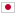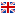Contest Duration: - (local time) (300 minutes) Back to Home
F - Point Sequences/Time Limit: 2 sec / Memory Limit: 1024 MB

Score : 100 points

### Problem Statement

Takahashi-kun sends integer sequences to Aoki-kun every year as his birthday present. But in this year, Takahashi-kun plans to send sequences of points on a two-dimensional plane to Aoki-kun.

Firstly, he makes 3 point sequences: (a_0, a_1, ..., a_{N-1}), (b_0, b_1, ..., b_{N-1}), and (c_0, c_1, ..., c_{N-1}), and makes a point d_0. Then he makes the points d_1, d_2, ..., d_N in this order as follows:

• For each i = 0, 1, ..., {N-1}, d_{i+1} is defined as the intersection point of two lines: the line that passes through a_i and b_i, and the line that passes through c_i and d_i.

It can happen that a point d_{i+1} can not be defined for some i. For example, when two lines are the same, there are an infinite number of intersection points.

Takahashi-kun wants to know the smallest i such that d_{i+1} can not be defined. To be precise, d_{i+1} can not be defined if either of the following conditions is met.

• c_i = d_i
• two lines given by a_i, b_i, c_i, and d_i are the same, or parallel.

It is guaranteed that a_i \neq b_i for all i.

Note that, in the following sections, x- and y- coordinates of a point p are denoted as p.x and p.y, respectively.

### Constraints

• 1 \leq N \leq 100,000
• |a_i.x|, |a_i.y|, |b_i.x|, |b_i.y|, |c_i.x|, |c_i.y| \leq 1,000
• |d_0.x|, |d_0.y| \leq 1000
• a_i \neq b_i
• All values in input are integers.

### Input

Input is given from Standard Input in the following format:

N
d_0.x d_0.y
a_0.x a_0.y b_0.x b_0.y c_0.x c_0.y
a_1.x a_1.y b_1.x b_1.y c_1.x c_1.y
:
a_{N-1}.x a_{N-1}.y b_{N-1}.x b_{N-1}.y c_{N-1}.x c_{N-1}.y


### Output

Print the smallest i such that d_{i+1} can not be defined. If all points are defined, print N.

### Sample Input 1

6
0 0
10 -10 10 10 10 0
0 10 20 10 10 10
0 -10 0 -100 0 10
0 0 0 -100 0 0
0 0 0 1 0 0
31 14 15 92 65 35


### Sample Output 1

3


### Sample Input 2

11
0 0
299 0 299 1 3 1
1 0 1 1 300 100
299 0 299 1 3 1
1 0 1 1 300 100
299 0 299 1 3 1
1 0 1 1 300 100
299 0 299 1 3 1
1 0 1 1 300 100
299 0 299 1 3 1
1 0 1 1 300 100
0 0 3 1 3 1


### Sample Output 2

10